# Methodologies for Performance Simulation of Superscalar OOO processors

• Slides: 32Methodologies for Performance Simulation of Super-scalar OOO processors Srinivas Neginhal Anantharaman Kalyanaraman Cpr. E 585: Survey Project 1Architectural Simulators n n n Explore Design Space Evaluate existing hardware, or Predict performance of proposed hardware Designer has control Functional Simulators: Performance Simulators: Model architecture (programmers’ focus) Model microarchitecture (designer’s focus) Eg. , sim-fast, sim-safe Eg. , cycle-by-cycle (sim-outoforder) 2Simulation Issues n n Real-applications take too long for a cycle-by-cycle simulation !! Vast design space: n Design Parameters: n n code properties, value prediction, dynamic instruction distance, basic block size, instruction fetch mechanisms, etc. Architectural metrics: n IPC/ILP, cache miss rate, branch prediction accuracy, etc. n Find design flaws + Provide design improvements n Correctness and accuracy of simulation results n Need a “fast and robust” simulation methodology !! 3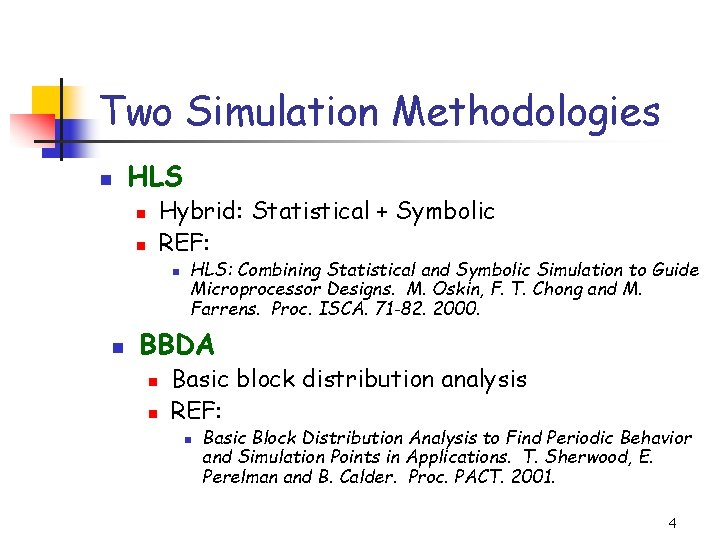Two Simulation Methodologies n HLS Hybrid: Statistical + Symbolic REF: n n HLS: Combining Statistical and Symbolic Simulation to Guide Microprocessor Designs. M. Oskin, F. T. Chong and M. Farrens. Proc. ISCA. 71 -82. 2000. BBDA n n Basic block distribution analysis REF: n Basic Block Distribution Analysis to Find Periodic Behavior and Simulation Points in Applications. T. Sherwood, E. Perelman and B. Calder. Proc. PACT. 2001. 4HLS: An Overview n A hybrid processor simulator Statistical Model HLS Symbolic Execution Performance Contours spanned by design space parameters What can be achieved? Explore design changes in architectures and compilers that would be impractical to simulate using conventional simulators 5HLS: Main Idea Synthetically generated code/data Large Application code Statistical Profiling Code characteristics: Architecture metrics: -basic block size -Cache behavior -Dynamic instruction distance -Branch prediction accuracy Instruction stream, data stream Structural Simulation of FU, issue pipeline units -Instruction mix sim-fast: Statistical Profiling sim-outorder: Structural Simulation 6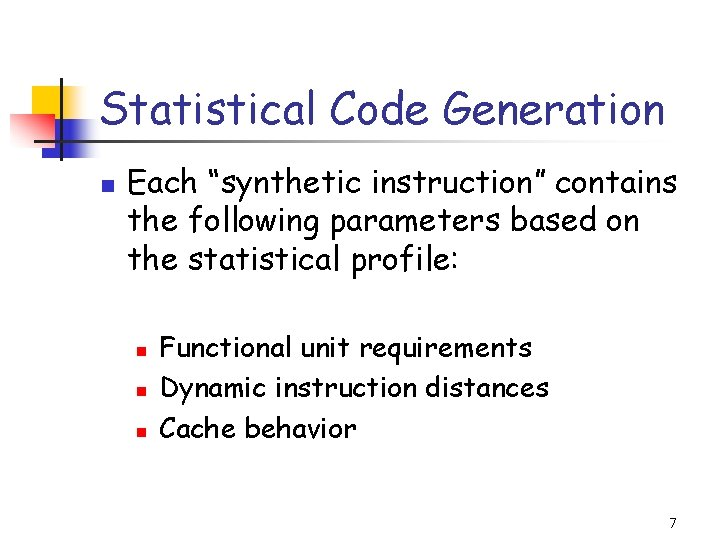Statistical Code Generation n Each “synthetic instruction” contains the following parameters based on the statistical profile: n n n Functional unit requirements Dynamic instruction distances Cache behavior 7HLS Correctness and Accuracy n n Validate HLS against Simple. Scalar (use IPC) For varying combinations of design parameters: n n n Run original benchmark code on Simple. Scalar (use sim-outoforder) Run statistically generated code on HLS Compare Simple. Scalar IPC vs. HLS IPC 8Validation: Single- and Multi-value correlations IPC vs. L 1 -cache hit rate For SPECint 95: HLS Errors are within 5 -7% of the cycle-by-cycle results !! 9HLS: Code Properties Basic Block Size vs. L 1 -Cache Hit Rate Inferred Correlation: Increasing basic block size helps only when L 1 cache hit rate is >96% or <82% 10HLS: Value Prediction GOAL: Break True Dependency DID vs. Value predictability Stall Penalty for mispredict vs. Value Prediction Knowledge 11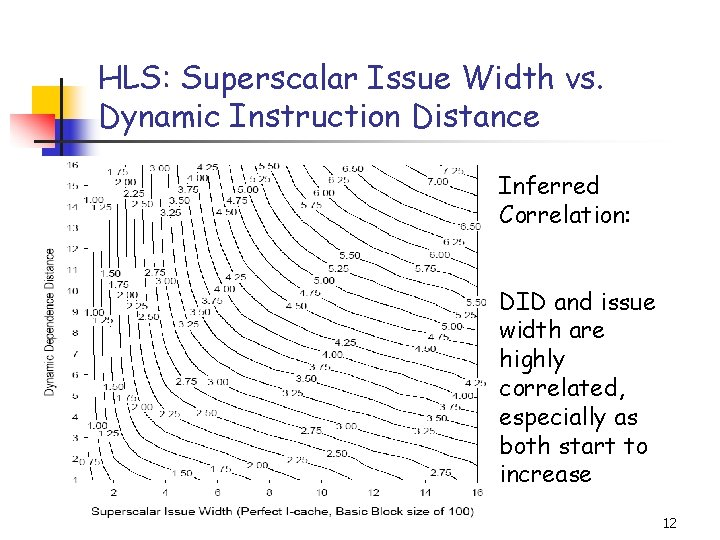HLS: Superscalar Issue Width vs. Dynamic Instruction Distance Inferred Correlation: DID and issue width are highly correlated, especially as both start to increase 12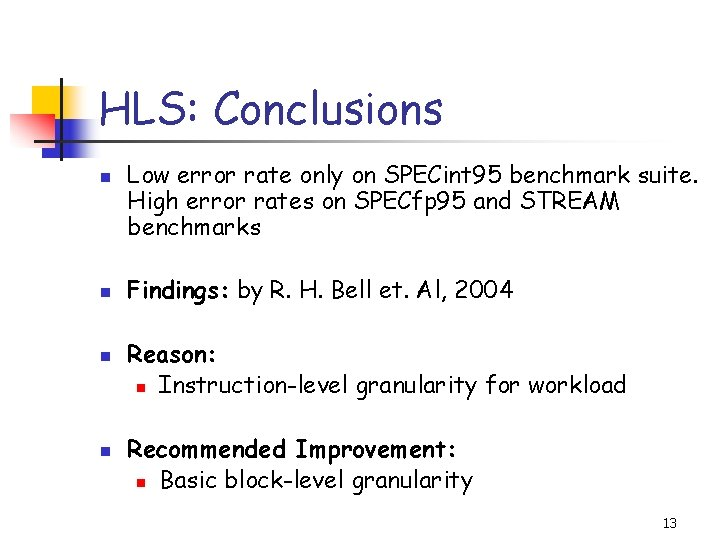HLS: Conclusions n n Low error rate only on SPECint 95 benchmark suite. High error rates on SPECfp 95 and STREAM benchmarks Findings: by R. H. Bell et. Al, 2004 Reason: n Instruction-level granularity for workload Recommended Improvement: n Basic block-level granularity 13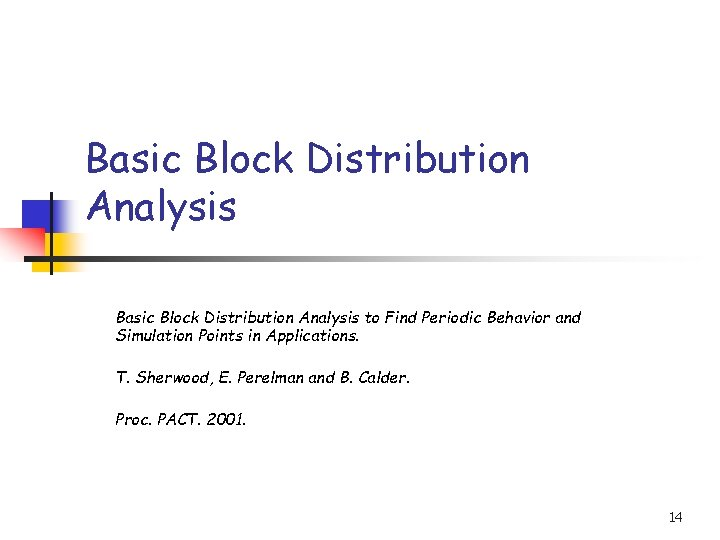Basic Block Distribution Analysis to Find Periodic Behavior and Simulation Points in Applications. T. Sherwood, E. Perelman and B. Calder. Proc. PACT. 2001. 14Introduction Goal n n To capture large scale program behavior in significantly reduced simulation time. Initialization Simulation Points Approach n n n Find a representative subset of the full program. Find an ideal place to simulate given a specific number of instructions one has to simulate Accurate confidence estimation of the simulation point. Period Program Execution n 15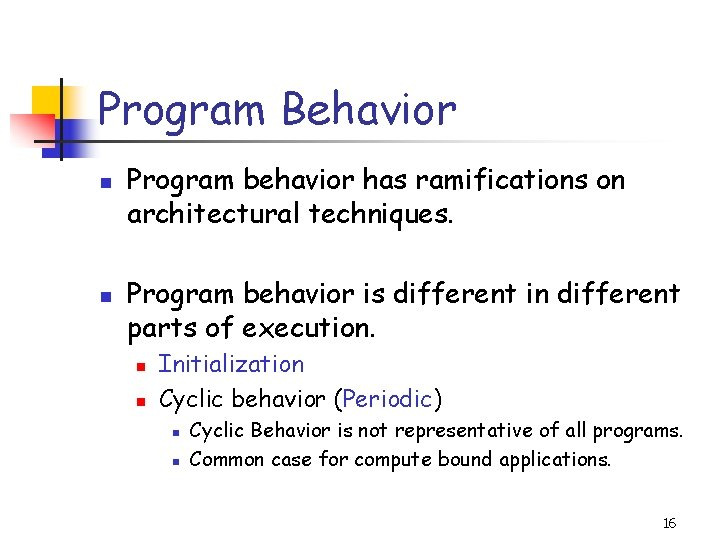Program Behavior n n Program behavior has ramifications on architectural techniques. Program behavior is different in different parts of execution. n n Initialization Cyclic behavior (Periodic) n n Cyclic Behavior is not representative of all programs. Common case for compute bound applications. 16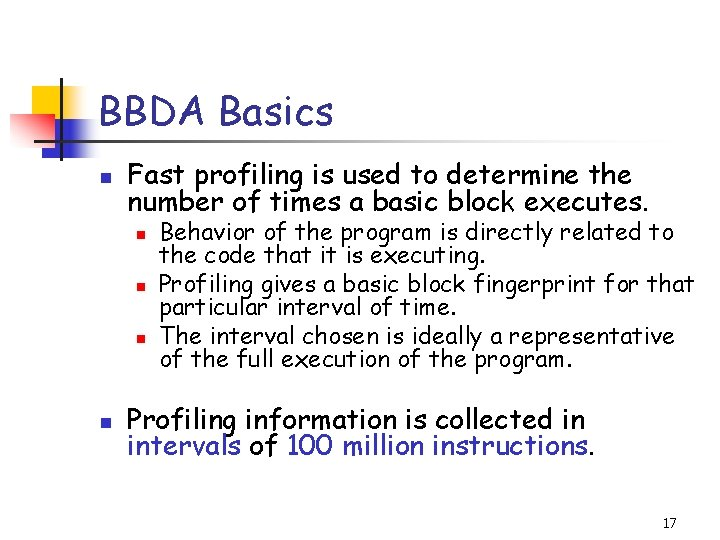BBDA Basics n Fast profiling is used to determine the number of times a basic block executes. n n Behavior of the program is directly related to the code that it is executing. Profiling gives a basic block fingerprint for that particular interval of time. The interval chosen is ideally a representative of the full execution of the program. Profiling information is collected in intervals of 100 million instructions. 17Basic Block Vector (BBV) BBV for Interval i: Interval i B 1 B 2 … Bx B 2 BD Frequency D: Total number of Basic blocks in the program code BBV = Fingerprint of an interval Varying size intervals A BBV collected over an interval of N times 100 million instructions is a BBV of duration N. 18Target BBV n BBVs are normalized n n Target BBV n n Each element divided by the sum of all elements. BBV for the entire execution of the program. Objective n Find a BBV of smallest duration “similar” to Target BBV. 19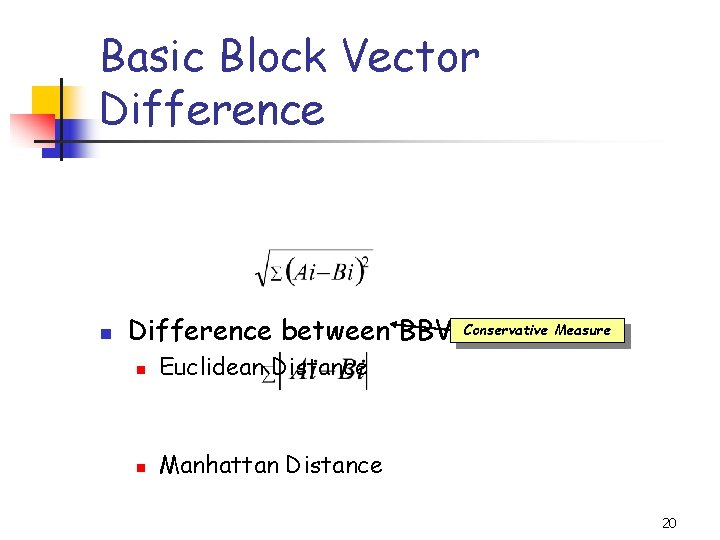Basic Block Vector Difference n Difference between BBVs. Conservative Measure n Euclidean Distance n Manhattan Distance 20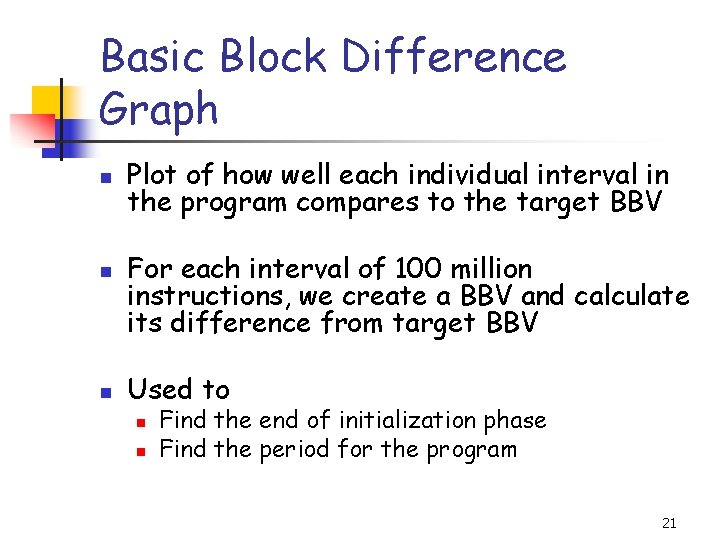Basic Block Difference Graph n n n Plot of how well each individual interval in the program compares to the target BBV For each interval of 100 million instructions, we create a BBV and calculate its difference from target BBV Used to n n Find the end of initialization phase Find the period for the program 21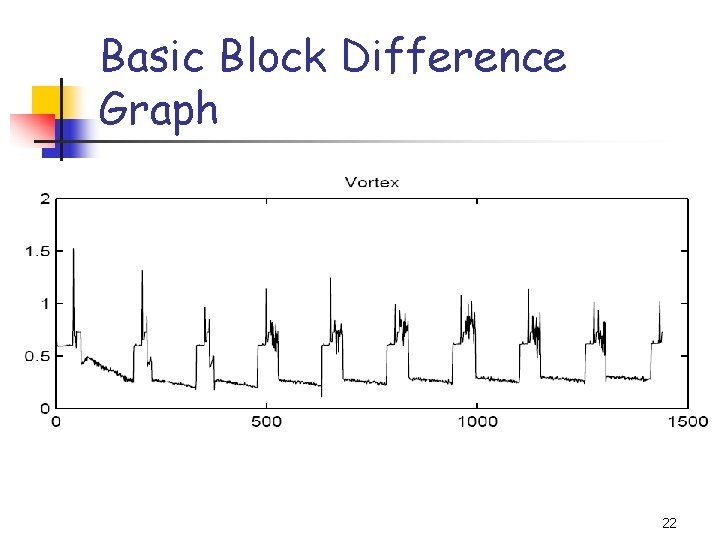Basic Block Difference Graph 22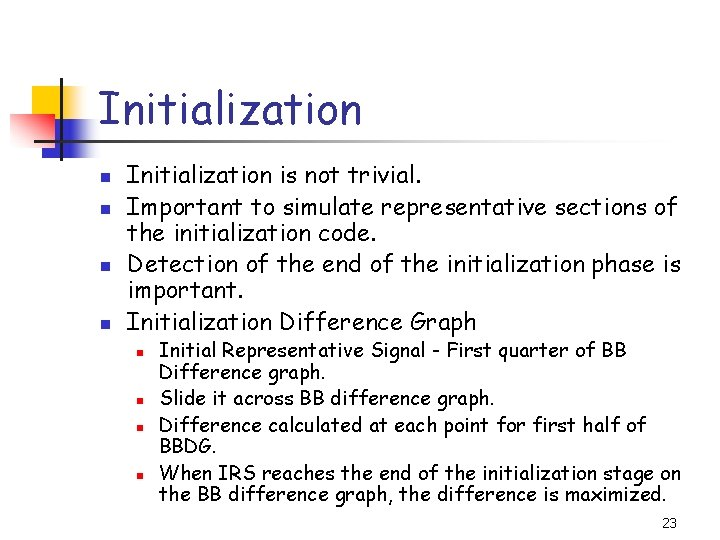Initialization n n Initialization is not trivial. Important to simulate representative sections of the initialization code. Detection of the end of the initialization phase is important. Initialization Difference Graph n n Initial Representative Signal - First quarter of BB Difference graph. Slide it across BB difference graph. Difference calculated at each point for first half of BBDG. When IRS reaches the end of the initialization stage on the BB difference graph, the difference is maximized. 23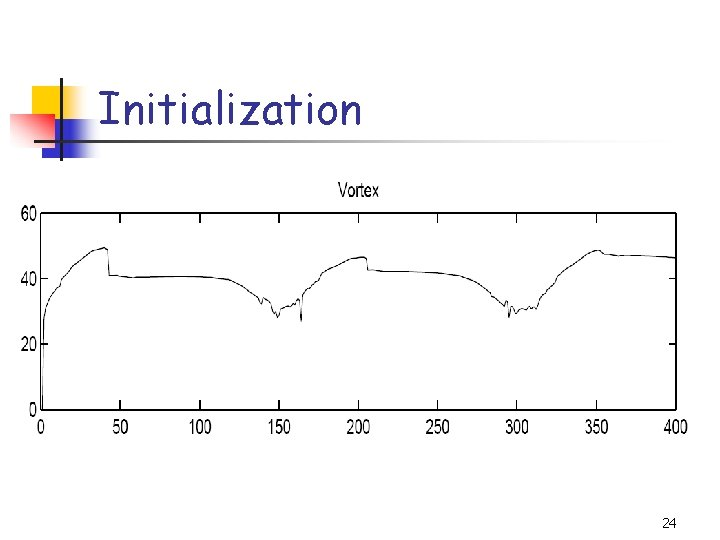Initialization 24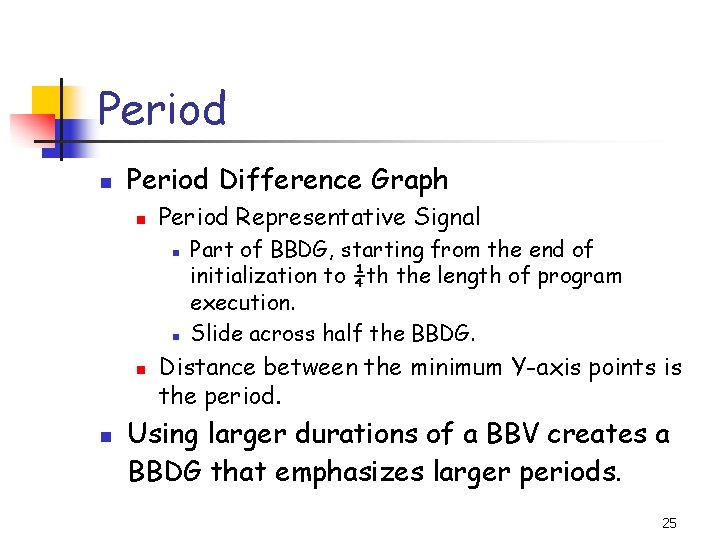Period n Period Difference Graph n Period Representative Signal n n Part of BBDG, starting from the end of initialization to ¼th the length of program execution. Slide across half the BBDG. Distance between the minimum Y-axis points is the period. Using larger durations of a BBV creates a BBDG that emphasizes larger periods. 25Period 26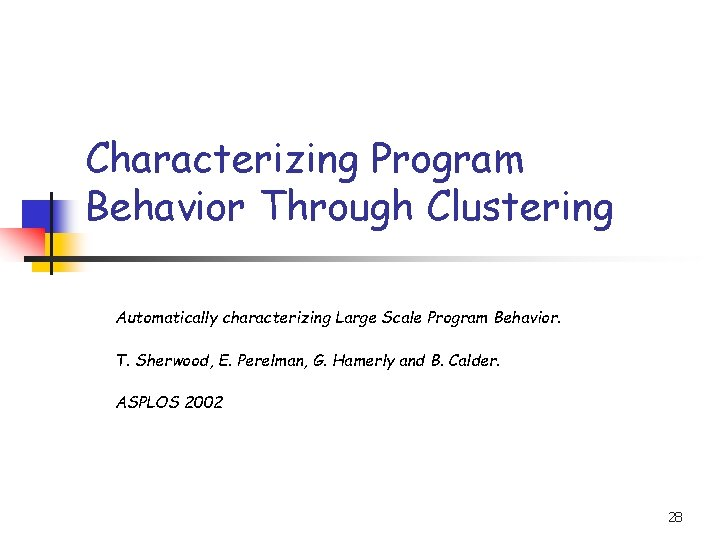Characterizing Program Behavior Through Clustering Automatically characterizing Large Scale Program Behavior. T. Sherwood, E. Perelman, G. Hamerly and B. Calder. ASPLOS 2002 28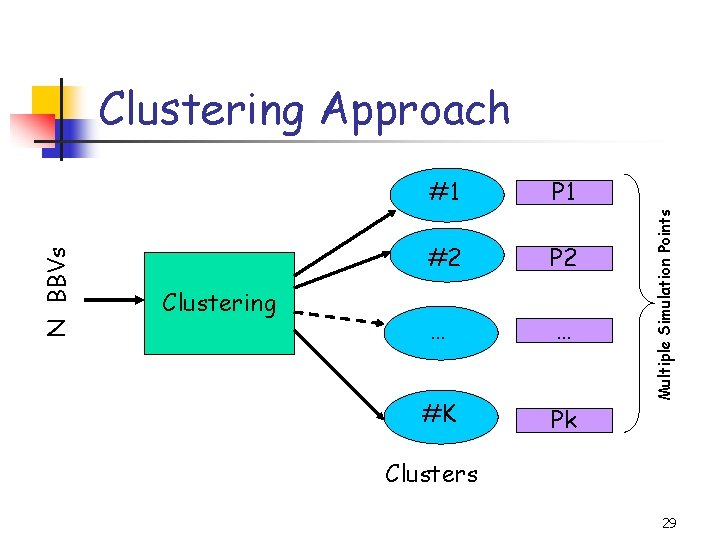Clustering #1 P 1 #2 P 2 … … #K Pk Multiple Simulation Points N BBVs Clustering Approach Clusters 29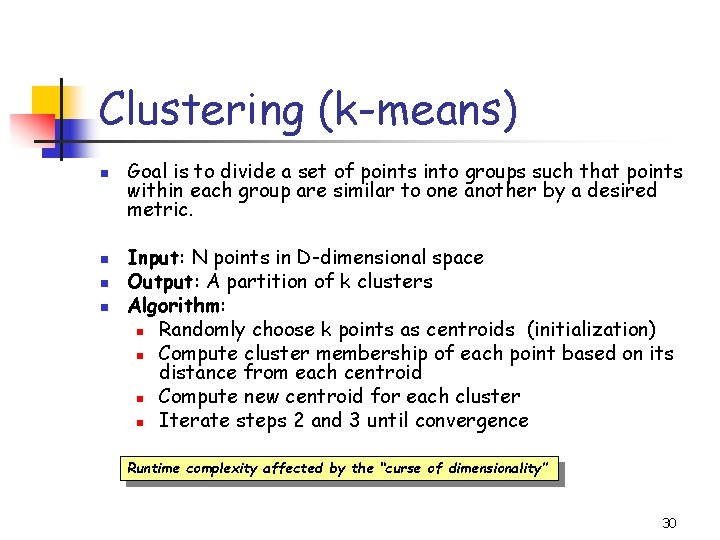Clustering (k-means) n n Goal is to divide a set of points into groups such that points within each group are similar to one another by a desired metric. Input: N points in D-dimensional space Output: A partition of k clusters Algorithm: n Randomly choose k points as centroids (initialization) n Compute cluster membership of each point based on its distance from each centroid n Compute new centroid for each cluster n Iterate steps 2 and 3 until convergence Runtime complexity affected by the “curse of dimensionality” 30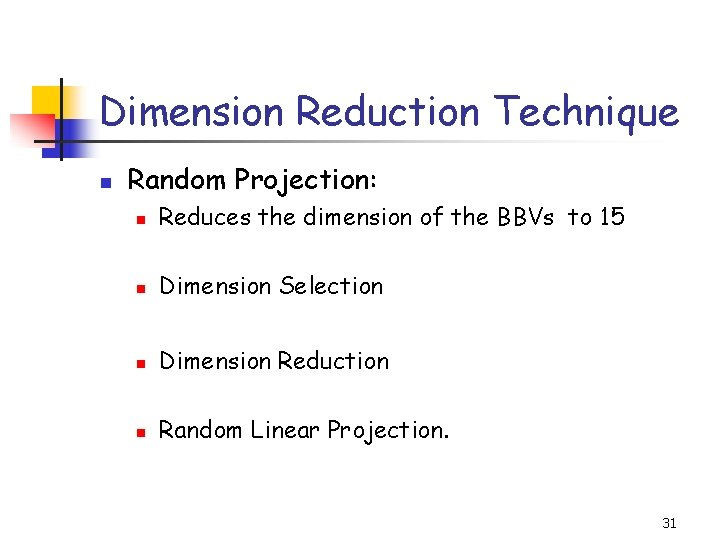Dimension Reduction Technique n Random Projection: n Reduces the dimension of the BBVs to 15 n Dimension Selection n Dimension Reduction n Random Linear Projection. 31BBDA: Conclusions n n n BBDA provides better sensitivity and lower performance variation in phases Other related work such as instruction working set technique provides higher “stability” For further evaluation of different techniques refer to n Comparing Program Phase Detection Techniques n A. S. Dhodapkar and J. E. Smith 32Related Work n Find smaller representative inputs: Klein Osowski et al. , 2000. n Fast forwarding and checkpointing: Haskins and Skadron, 2002. n Simulation points based: Lafage et al. , 2000. n Statistical Simulation: Oskin et al. , 2000. n Trace-driven approach for Statistical Simulation: Carl et al. , 1998. 33